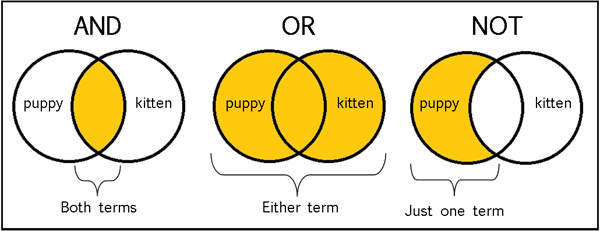Related Tags

pascal

# What are logical operators in Pascal expressions?Sarvech Qadir

In this shot, let’s talk about logical operators in the Pascal programming language. We often use these Boolean operands in condition variables.

 Operator How does it work? Condition Statement and This refers to the AND operator often used in boolean calculations. It gives output True if both inputs A and B are True. Output = True IF (A = True and B = True) or This refers to the OR operator often used in boolean calculations. It gives output True if any of the inputs A or B are True. Output = True IF (A = True or B = True)Output = True IF (A = True or B = False)Output = True IF (A = False or B = True) not This refers to the OR operator often used in boolean calculations. It is used to reverse the initial value of any input. output = False if not Aoutput = False if not Boutput = true if (not A or B)Logical Operators

## Code

Let’s look at the code for all of these logical operators.

program beLogical;
var a, b : boolean;

begin
a := true;
b := true;
// applying AND
if (a and b) then
writeln('Condition AND is True')

else
writeln('Condition AND is False');
// applying OR
if  (a or b) then
writeln('OR condition is True')

else
writeln('OR condition is False');
// applying OR
writeln('Applying NOT on input a = ',not a);

// Changing values a little
a := true;
b := False;
if (a and b) then
writeln('Condition AND is True')
else
writeln('Condition AND is False');
if  (a or b) then
writeln('OR condition is True')
else
writeln('OR condition is False');

writeln('Applying NOT on input b = ',not b);

end.


### Output:

Condition AND is True
OR condition is True
Applying NOT on input a = FALSE
Condition AND is False
OR condition is True
Applying NOT on input b = TRUE


RELATED TAGS

pascal

CONTRIBUTORSarvech Qadir